## Sunday, February 23, 2020

### Some non-convex quadratic models I

The new version of Gurobi v 9 introduced support for non-convex quadratic models. Cplex already supported some classes of such models (such as non-convex QPs). Here are just some models we can now try to solve with these solvers.

It is noted that you need to specify a solver option for Cplex and Gurobi to accept most non-convex models, Some models can be reformulated by the solver, but "real" con-convex models require the option OptimalityTarget 3 (Cplex) or NonConvex 2 (Gurobi).

#### 1a. Largest Empty Rectangle

Given is an area with $$n$$ points:n=50 random points

The problem is to find the largest empty rectangle, i.e., without any points inside. The solution looks like: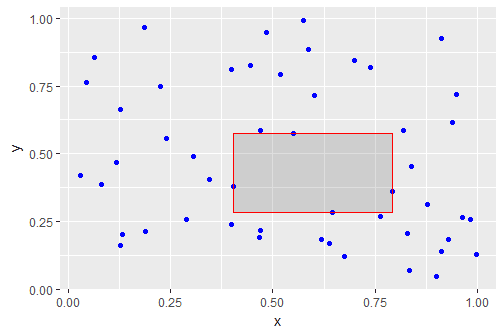Largest empty rectangle

The idea behind the model below, it that a data point $$p_i$$ must be either left, right, below or above the rectangle. So it the coordinates of the rectangle are denoted by $$r_{x,lo},r_{x,up},r_{y_lo},r_{y_up}$$, then at least one of the following constraints must hold: \begin{align} & p_{i,x} \le r_{x,lo} \\& p_{i,y} \le r_{y,lo} \\ & p_{i,x} \ge r_{x,up} \\& p_{i,y} \ge r_{y,up}\end{align} The complete mathematical model to find this rectangle can be stated as:

Smallest empty rectangle
\begin{align} \max\> & \color{darkred}{\mathit{area}} = \prod_c (\color{darkred}r_{c,up}-\color{darkred}r_{c,lo}) \\ & \color{darkblue}p_{i,c} \le \color{darkred}r_{c,lo}+\color{darkblue}M_c(1-\color{darkred}\delta_{i,c,lo}) && \forall i,c\\ & \color{darkblue}p_{i,c} \ge \color{darkred}r_{c,up}-\color{darkblue}M_c(1-\color{darkred}\delta_{i,c,up}) && \forall i,c\\ & \sum_{c,s} \color{darkred}\delta_{i,c,s} \ge 1 && \forall i \\ & \color{darkblue} L_c \le \color{darkred}r_{c,lo} \le \color{darkred}r_{c,up}\le \color{darkblue} U_c \\ & \color{darkred}\delta_{i,c,s} \in \{0,1\} \end{align}
\begin{align} & i = \{1,\dots,n\}\\ & c = \{x,y\}\\ & s = \{lo,up\} \\ & \color{darkblue} L_c = 0 \\ & \color{darkblue} U_c = 1 \\ & \color{darkblue} M_c = \color{darkblue}U_c-\color{darkblue}L_c \end{align}

For the 2d case, the objective can be written as $\min\> \mathit{area} = (r_{x,up}-r_{x,lo})\cdot (r_{y,up}-r_{y,lo})$ The outer area is a given rectangle with limits $$L_x, U_x, L_y, U_x$$. I used here the unit box: $$L_x=L_y = 0$$ and $$U_x=U_y = 1$$. The big-M constants $$M_x, M_y$$ need tight values. We can use the area dimensions for that. For a formulation without big-M constraints, we can implement implications using indicator constraints: \begin{align} & \delta_{i,c,lo}=1 \implies p_{i,c} \le r_{i,c,lo} \\ &\delta_{i,c,up}=1 \implies p_{i,c} \ge r_{i,c,up}\end{align}

The problem is not convex because of the objective. It is noted that we can simplify the objective a bit by writing \begin{align}\max\>&\mathit{area} = \mathit{side}_x \cdot \mathit{side}_y\\ & \mathit{side}_c = r_{c,up} - r_{c,lo}\end{align} This is a small problem with just 4 continuous quadratic variables and $$n\times 4$$ binary variables. Gurobi or Cplex solve this model very quickly.

This problem can also be solved with available global solvers. Both Antigone and Baron have no problems with it. The optimal solution looks like:

----     50 VARIABLE area.L                =        0.113

----     50 VARIABLE r.L  coordinates of rectangle

lo          up

x       0.403       0.792
y       0.285       0.575


I also tried the open-source solver Couenne. That was a surprise:

               S O L V E      S U M M A R Y

MODEL   m                   OBJECTIVE  area
TYPE    MIQCP               DIRECTION  MAXIMIZE
SOLVER  COUENNE             FROM LINE  49

**** SOLVER STATUS     1 Normal Completion
**** MODEL STATUS      1 Optimal
**** OBJECTIVE VALUE                0.0121

----     50 VARIABLE area.L                =        0.012

----     50 VARIABLE r.L  coordinates of rectangle

lo          up

x       0.939       0.982
y       0.720       1.000


This is obviously a bad and non-optimal solution. I don't know what is going on here, but this looks like a bug.

#### 1b. 3d largest box

It is easy to make a 3d version of the model above. Just replace $$c = \{x,y\}$$ by $$c = \{x,y,z\}$$. Unfortunately, there is one complication: now the model has an objective that is no longer quadratic. However, this can be fixed by chaining: \begin{align} \max\>&\mathit{volume} = \mathit{area}\cdot \mathit{side}_{z}\\ & \mathit{area} = \mathit{side}_{x} \cdot \mathit{side}_{y} \\ & \mathit{side}_c = r_{c,up}-r_{c,lo} && \forall c \end{align}

A solution can look like:

----     52 VARIABLE area.L                =        0.340
VARIABLE volume.L              =        0.146

----     52 VARIABLE r.L  coordinates of 3D box

lo          up

x       0.608       0.998
y       0.091       0.963
z       0.140       0.571


Note that Cplex can not handle this problem: Cplex allows non-convexities in the objective only (apart for some cases where it can reformulate things).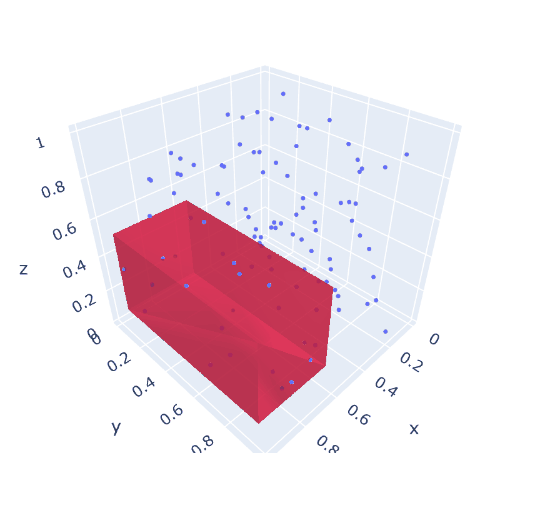3d version: largest box not clashing with given points

#### 2. Hostile Brothers Problem

A father is facing the following problem. He has has a plot of land. And he has $$n$$ sons, who don't get along at all. In order to minimize friction, he wants to build a house for each of his sons, such that the minimum distance between them is maximized. The mathematical model can look like:

Hostile Brothers Problem
\begin{align} \max\> & \color{darkred}z \\ & \color{darkred}z \le \sum_{c} (\color{darkred}x_{i,c}-\color{darkred}x_{j,c})^2&& \forall i\lt j\\ & \color{darkred}x_{i,c} \in [\color{darkblue}L,\color{darkblue}U]\end{align}

Here $$i$$ indicates the point, $$i \in \{1,\dots,n\}$$ and $$c$$ is the coordinate. For a 2D problem, we have $$c \in \{x,y\}$$.

When we use $$n=50$$ random points, Gurobi is not able to even come close to proven optimality, I used a time limit of 2 hours, and the final gap is enormous:

 1560307 1354785    0.40487   42 1653    0.01935    0.67173  3371%   332 7171s
1562085 1356368     cutoff  283         0.01935    0.67173  3371%   332 7177s
1563023 1356980     cutoff  285         0.01935    0.67173  3371%   332 7180s
1564565 1358071    0.01937  248  930    0.01935    0.67173  3371%   332 7190s
1565720 1359205    0.60071   48 1510    0.01935    0.67173  3371%   332 7196s
1567335 1359826     cutoff  272         0.01935    0.67173  3371%   332 7200s

Cutting planes:
RLT: 1312

Explored 1567341 nodes (520860935 simplex iterations) in 7200.00 seconds
Thread count was 8 (of 16 available processors)


Summary:

• Best found solution: 0.01935
• Best possible objective value: 0.6173
• Relative gap: 3371%

The big gap is partly due to the small best-found solution (0.01935). But mainly: this is a very difficult job for Gurobi. Even when the problem is relatively small: just 100 continuous variables,

By inspecting the solution, we see this solution is not very good at all:Solution found by Gurobi after 2 hours (obj=0.01935)

I expect a much more regular pattern.

We can try to improve performance by noticing there is much symmetry in the model. The same solution can be obtained by just renumbering the points. To break symmetry, I added the constraint: $\sum_c x_{i,c} \ge \sum_c x_{i-1,c}$ This was not very successful:

 2978972 2407171    0.01708  130  813    0.00885    0.64271  7159%   222 7180s
2981109 2409232    0.52720   64 2378    0.00885    0.64271  7159%   222 7185s
2984648 2411500    0.20399  107 2156    0.00885    0.64271  7159%   222 7191s
2986302 2412811    0.12220  127 1721    0.00885    0.64271  7159%   222 7195s
2987852 2413879    0.06942  175 1728    0.00885    0.64271  7159%   222 7200s

Explored 2988184 nodes (662244035 simplex iterations) in 7200.01 seconds
Thread count was 8 (of 16 available processors)


So we see:
• The best-found solution (0.00885) is worse than before (0.01935)
• The best possible solution (0.64271) is worse than before (0.6173)
• The relative gap is worse (7159% vs 3371%)
As an alternative, let's try Baron on this model. I dropped the symmetry breaking constraint here (adding this constraint was not beneficial, at least not during the initial period of the search). We see in the first few minutes:

  Iteration    Open nodes         Time (s)    Lower bound      Upper bound
*         1             1             5.51     0.257746E-01     2.00000
*         1             1             7.22     0.274782E-01     2.00000
1             1             7.41     0.274782E-01     2.00000
12             7            49.51     0.274782E-01     1.72727
31            16            80.03     0.274782E-01     1.72727
46            24           111.27     0.274782E-01     1.72727


I.e. Baron finds a much better solution very quickly (but is far from getting the gap close to zero).

Another approach is to use a multi-start approach with a local NLP solver. Generate a random starting point, solve, and repeat. When I do this 20, I see when I use the CONOPT NLP solver:

----     57 PARAMETER objs  objective values of NLP solver

cycle1  0.02751,    cycle2  0.02669,    cycle3  0.02705,    cycle4  0.02041,    cycle5  0.02620,    cycle6  0.01235
cycle7  0.02675,    cycle8  0.02590,    cycle9  0.02660,    cycle10 0.02679,    cycle11 0.02558,    cycle12 0.02644
cycle13 0.02622,    cycle14 0.02625,    cycle15 0.02620,    cycle16 0.02749,    cycle17 0.02742,    cycle18 0.02692
cycle19 0.02675,    cycle20 0.02688,    best    0.02751


This is the best we have seen so far. By accident, the best results were found in the first cycle. The results look like:Best solution with Multi-start Conopt (obj=0.02751)

This solution looks much better than the one Gurobi found. In addition, CONOPT needs only 30 seconds to solve these 20 problems.

Conclusion:

• Cplex cannot solve this problem
• Gurobi finds a solution with objective 0.01935 (time limit: 2 hours)
• Baron finds a solution of  0.02748 in 100 seconds
• Conopt finds a solution of 0.02751 in a loop of 20 solves that takes 30 seconds
• Increasing this to 200 solves gives an objective of 0.02771
• Remember that we are maximizing
Even though this is a non-convex quadratic problem, it makes sense to look beyond quadratic solvers.

## Monday, February 10, 2020

### Longest path problem

This is a question that regularly pops up: how to solve the longest path problem? At first sight, this is easy. Just replace the "minimize" in the shortest path problem by "maximize" and we are good to go. Unfortunately, opposed to the well-known shortest path problem, the longest path problem is not that easy to state and to solve.

#### 1. Standard Shortest Path Formulation

The standard shortest path can be formulated as a Mixed Integer Programming problem:

Shortest Path Problem
\begin{align} \min& \sum_{(i,j)\in\color{darkblue} A} \color{darkblue}d_{i,j} \color{darkred}x_{i,j} \\ & \sum_{j|(j,i)\in \color{darkblue}A} \color{darkred}x_{j,i} + \color{darkblue}b_i = \sum_{j|(i,j)\in \color{darkblue}A} \color{darkred}x_{i,j} && \forall i \\ &\color{darkred}x_{i,j} \in \{0,1\} \\ & \color{darkblue} b_i = \begin{cases}1 & \text{if i is a source node} \\ -1 & \text{if i is a sink node} \\ 0 & \text{otherwise} \end{cases} \end{align}

where $$A$$ is our network and $$b$$ is a sparse data vector indicating the exogenous inflow or outflow at the source or sink node.Network with the shortest path

There are some implicit assumptions in this model: there are no negative cycles. In the above example, $$d_{i,j}$$ represents distance, so we have $$d_{i,j}\ge 0$$ and there is no problem of negative cycles.

Shortest path problems are very easy MIPs to solve. The solution is automatically integer, so it can be solved as an LP and these LPs are very sparse (only two non-zero elements per column) and very easy to solve. In addition, specialized network solvers are widely available.

The problem in the picture has 20 nodes and 37 × 2 arcs. In this example, the arcs are duplicated to make sure we can go in two directions. This leads to an LP of 74 variables and 20 constraints. The solution looks like:

----     75 VARIABLE z.L                   =      128.538  objective

----     75 VARIABLE x.L  flow

point6(B)     point15     point16     point18     point20

point5(A)                               1.000
point15         1.000
point16                                             1.000
point18                                                         1.000
point20                     1.000


#### 2. Just use max

When we just turn the above problem in a maximization problem, we get results that are difficult to interpret. But they certainly don't form what we would call a path:

----     78 VARIABLE z.L                   =     1638.406  objective

----     78 VARIABLE x.L  flow

point1      point2      point3      point4   point5(A)   point6(B)      point7      point8      point9

point1                                              1.000
point3                                                                                                          1.000
point4          1.000
point5(A)                                                                                           1.000
point6(B)                                                                               1.000
point7                                                                      1.000
point8                                                          1.000
point9                                  1.000
point10                     1.000
point12                                                                                                         1.000
point13                                                                                 1.000
point14                                             1.000                                           1.000
point15                                                                     1.000
point16                                                                                             1.000
point17         1.000                               1.000
point18                                 1.000                                                                   1.000
point19                                                                                 1.000
point20                     1.000

+     point10     point11     point12     point13     point14     point15     point16     point17     point18

point1                                                                                              1.000
point2          1.000
point3                                                                                                          1.000
point4                                                          1.000                               1.000
point5(A)                                                                               1.000
point7                                              1.000
point8                                                          1.000                   1.000
point9                                  1.000                                                                   1.000
point10                                                                     1.000
point11                                                                                                         1.000
point12                                                                                 1.000                   1.000
point14                                                                                 1.000       1.000
point15         1.000
point16                                 1.000                   1.000                               1.000       1.000
point17                                                         1.000                   1.000                   1.000
point18                     1.000       1.000                                                       1.000
point19                                             1.000                   1.000                   1.000       1.000
point20         1.000       1.000                   1.000                   1.000

+     point19     point20

point2                      1.000
point7          1.000
point10                     1.000
point11                     1.000
point13         1.000       1.000
point15         1.000
point17         1.000
point18         1.000       1.000
point19                     1.000
point20         1.000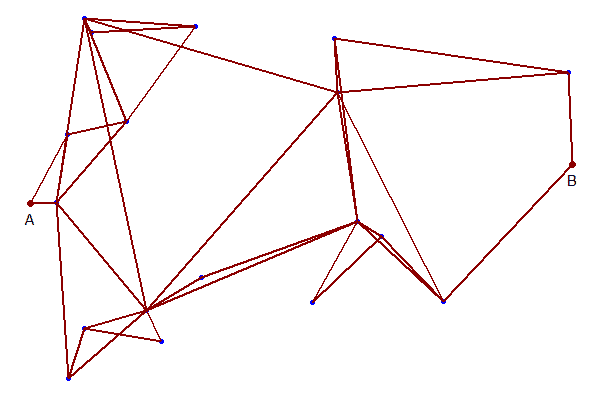Maximization results

Basically, all but a few lines in the plot are traveled in both directions. Conclusion: this is not what we are looking for.

#### 3. TSP-like solution

To form a proper path (called an elementary path), we need to add two sets of constraints :
1. The outflow of each node goes to just one arc: $\sum_{j|(i,j)\in A} x_{i,j} \le 1\>\forall i$
2. Forbid any sub-tours to be formed. Sub-tour elimination constraints are well-known from the Traveling Salesman Problem.
Using the simple MTZ (Miller, Tucker, and Zemlin) approach, we can formulate:

Longest Path Problem
\begin{align} \max& \sum_{(i,j)\in\color{darkblue} A} \color{darkblue}d_{i,j} \color{darkred}x_{i,j} \\ & \sum_{j|(j,i)\in \color{darkblue}A} \color{darkred}x_{j,i} + \color{darkblue}b_i = \sum_{j|(i,j)\in \color{darkblue}A} \color{darkred}x_{i,j} && \forall i \\ & \sum_{j|(i,j)\in \color{darkblue}A} \color{darkred}x_{i,j} \le 1 && \forall i\\ & \color{darkred}t_j \ge \color{darkred}t_i + 1 + (\color{darkblue}n-1)(\color{darkred}x_{i,j}-1) && \forall i,j|i\ne\text{source},j\ne\text{sink} \\&\color{darkred}x_{i,j} \in \{0,1\} \\ & \color{darkred}t_i \ge 0 \end{align}

Here $$n$$ is the number of nodes.

The results look like:

----    126 VARIABLE z.L                   =      432.987  objective

----    126 VARIABLE x.L  flow

point1      point2      point3      point4   point6(B)      point7      point8      point9     point10     point12

point2                                                                                                          1.000
point4          1.000
point5(A)                                                                               1.000
point9                                  1.000
point12                                                                                             1.000
point14                                             1.000
point15                                                         1.000
point16                                                                                                                     1.000
point19                                                                     1.000
point20                     1.000

+     point13     point14     point15     point16     point17     point18     point19     point20

point1                                                          1.000
point3                                                                      1.000
point7          1.000
point8                      1.000
point10                                 1.000
point13                                                                                             1.000
point17                                             1.000
point18                                                                                 1.000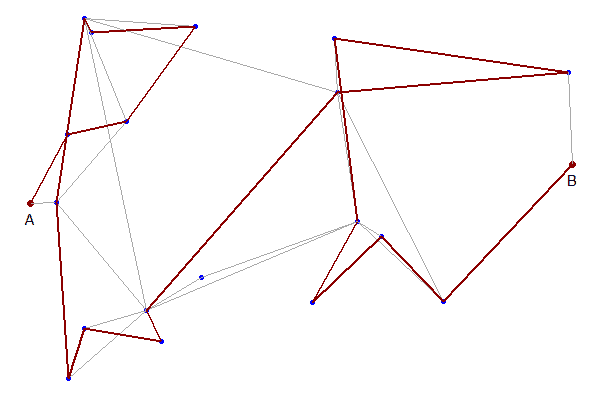Solution of Longest Elementary Path Model

This looks much better.

More formulations can be found in .

Let's drop the sub-tour elimination constraints, and have a look at the solution of the remaining model:

Problem A: drop sub-tour elimination constraints
\begin{align} \max& \sum_{(i,j)\in\color{darkblue} A} \color{darkblue}d_{i,j} \color{darkred}x_{i,j} \\ & \sum_{j|(j,i)\in \color{darkblue}A} \color{darkred}x_{j,i} + \color{darkblue}b_i = \sum_{j|(i,j)\in \color{darkblue}A} \color{darkred}x_{i,j} && \forall i \\ & \sum_{j|(i,j)\in \color{darkblue}A} \color{darkred}x_{i,j} \le 1 && \forall i\\ &\color{darkred}x_{i,j} \in \{0,1\} \end{align}

When we look at the solution we see a number of sub-tours of just two points. This means: travel from $$C\rightarrow D$$ and back.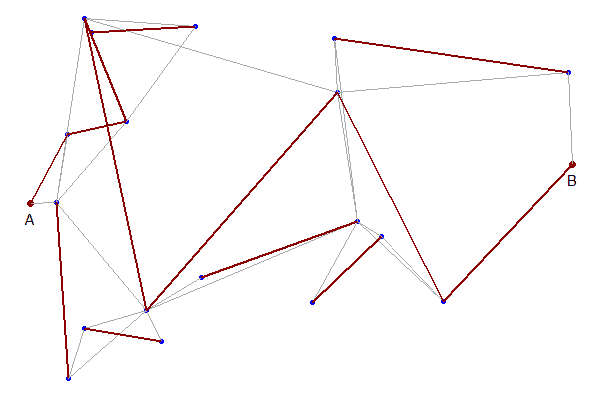Sub-tours with 2 points

These special sub-tours are easily prevented: add a cut of the form $x_{i,j}+x_{j,i} \le 1$ We can add them only for the $$i \rightarrow j \rightarrow i$$ offenders or just for all possible $$i \lt j$$. Here I did the last thing. The model becomes:

\begin{align} \max& \sum_{(i,j)\in\color{darkblue} A} \color{darkblue}d_{i,j} \color{darkred}x_{i,j} \\ & \sum_{j|(j,i)\in \color{darkblue}A} \color{darkred}x_{j,i} + \color{darkblue}b_i = \sum_{j|(i,j)\in \color{darkblue}A} \color{darkred}x_{i,j} && \forall i \\ & \sum_{j|(i,j)\in \color{darkblue}A} \color{darkred}x_{i,j} \le 1 && \forall i\\ & \color{darkred}x_{i,j}+\color{darkred}x_{j,i} \le 1 && \forall i \lt j \\ &\color{darkred}x_{i,j} \in \{0,1\} \end{align}

After adding these cuts and solving problem B we see:Results after adding cuts

The solution has no new sub-tours, so we can conclude this is the optimal solution.

Thus far this is a bit of an ad-hoc method. This approach would fail if we observe more complex sub-tours. However, it is not too difficult to make this more general. We can add appropriate cuts when we observe sub-tours and resolve the model. Such a cutting plane algorithm can actually outperform models where we add sub-tour elimination constraints in advance. With modern solvers, it is even possible to add this cut generation inside the branch-and-bound search (i.e. no resolve needed).

#### Conclusion

The conclusion is: "solve the longest path problem" is not as easy as it seems. We need to employ TSP-like machinery to solve this problem. That means no easy MIP models. Straight MIP models are both not that simple to formulate and will only work for relatively small models. A cutting plane approach can help here.

#### References

1. Leonardo Taccari, Integer programming formulations for the elementary shortest path problem, European Journal of Operational Research, Volume 252, Issue 1, 1 July 2016, Pages 122-130